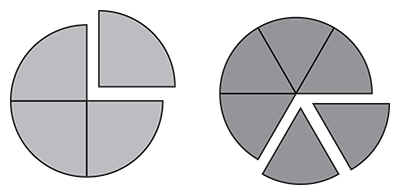Representing Fractions
Equivalent Fractions
Word Problems
Making Fraction
Find one Equivalent Fraction
100

What fraction does this picture represents?2/4

100

1/2 = ? /10

5

100

5/8 of the staff are male. 5/12 of the staff works part time at the aquarium. What fraction of the staff is female?

What is 3/8?

100

Find the fraction:

A cube has 6 square faces. One of them is shaded. Represent the shaded part in a fraction?

1/6

100

2/3=

100

Example; 4/8 + 1/8 = 5/8

Find 3/6+2/6

5/6

200

Which fraction is shown below?D) 1/2

200

3/4 = 12/?

16

200

The penguin nursery is open two times a day: 1/3 hour at noon and 5/12 hour in the afternoon. How much time is the penguin nursery open every day?

What is 9/12 or 3/4?
200

A cube has 6 square faces. One of them is shaded. Represent the not shaded parts of the cube using a fraction form.

5/6

200

10/20=

200

Example; 4/8 + 1/8 = 5/8

6/7 + 5/7

11/7

300

State the two fractions represented below.First image 1/4

Second image 2/6

300

7/8 = 14/?

16

300

An octopus weighed 5 6 kilograms. After two weeks, its weight was increased by 3 10 kilograms. But afterwards, it lost 1 5 kilograms in weight as it was sick. What is its current weight?

What is 14/15 kilograms?

300

In a box of 12 crayons, 4 are red color, the rest assorted. Write the fraction of red crayons in this box.

4/12

300

5/7=

300

Example; 4/8 + 1/8 = 5/8

1/8 + 3/8 =4/8

4/8

400

Which fraction is represented below?D) 3/8

400

18/20 = 9/?

10

400

The coffee cups can hold 7 9 of a pint of liquid. If Emily pours 2/3 of a pint of coffee into a cup, how much milk can a customer add?

What is 1/9?

400

In a box of 12 crayons, 4 are red color, the rest assorted. Write the fraction assorted crayons in this box.

8/12

400

50/120=

400

Example; 4/8 + 1/8 = 5/8

2/5 +3/5 =

5/5 or 1 whole

500

Identify the fraction below?D) 2/10

500

35/80 = ?/16

7

500

There were two cheesecakes in the fridge. The first cheesecake was cut into 16 slices and there are 5 slices left. The other cheesecake was cut into 8 slices and 6 slices were sold. How much cake is left in the fridge?

What is 15/16?

500

Ruth needs 3/4 cups of flour for one cake. She needs  the same amount of cups of flour for another cake, but for the last cake she needs to divide the flour into 8 equal part. Write the fraction of Ruth's second cake' flour.

500

36/70=